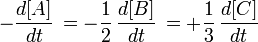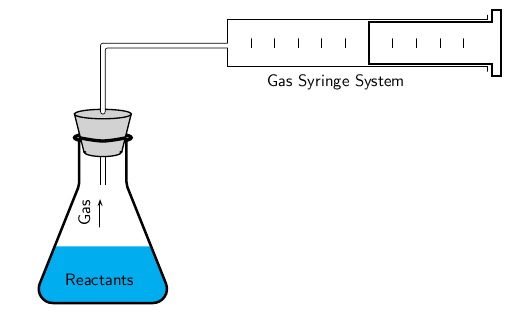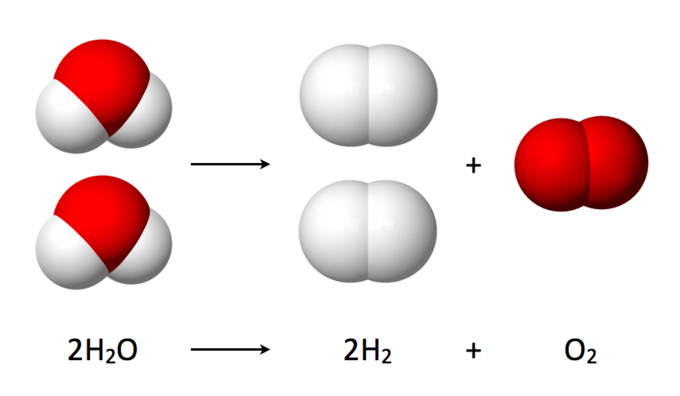Measuring Reaction Rates

Reaction rates are determined by observing the changes in the concentrations of reactants or products over a specific time frame.

Learning Objectives

Produce rate expressions when given chemical reactions and discuss methods for measuring those rates

Key Takeaways

Key Points

• Reaction rate is calculated using the formula rate = Δ[C]/Δt, where Δ[C] is the change in product concentration during time period Δt.
• The rate of reaction can be observed by watching the disappearance of a reactant or the appearance of a product over time.
• If a reaction produces a gas such as oxygen or carbon dioxide, there are two ways to measure the reaction rate: using a gas syringe to measure the gas produced, or calculating the reduction in the mass of the reaction solution.
• If the reaction produces a precipitate, the amount formed can be used to determine reaction rate by measuring how long it takes for the forming precipitate to obscure the visibility of a cross through a conical flask.

Key Terms

• reaction rate: How fast or slowly a reaction takes place.
• gas syringe: An item of laboratory equipment used to withdraw a volume of gas from a closed chemical system for measurement and/or analysis.
• product: A chemical substance formed as a result of a chemical reaction.

Reaction Rate

The rate of a reaction is usually observed by watching the disappearance of a reactant or the appearance of a product within a given time period. Take the chemical reaction:

$\text{A} + 2\text{B} \rightarrow 3\text{C}$

Here, the rate of appearance of product C in time interval Δt is:

$\text{average rate}=\frac{\Delta \text{C}}{\Delta \text{t}}$

The concentration of C, [C], is usually expressed in moles/liter. This is the average rate of appearance of C during the time interval Δt. The limit of this average rate as the time interval becomes smaller is called the rate of appearance of C at time t, and it is the slope of the curve of [C] versus t at time t. This instantaneous slope, or rate, is written $\frac{\text{d}[\text{C}]}{\text{dt}}$. Since one molecule of A and two molecules of B are consumed for every three molecules of C that are produced, the rates of disappearance and appearance of these chemical species are different, but related.Rates of disappearance and appearance of chemical species: This expression relates the rates of disappearance and appearance of chemical species in the reaction A + 2B –> 3C.

Measuring Reaction Rate

How the rate of a reaction is measured will depend on what the reaction is and what product forms. The following examples describe various ways to measure the rate of a reaction.

Reactions That Produce Gases Such as Oxygen or Carbon Dioxide

Hydrogen peroxide decomposes to produce oxygen:

$2\text{H}_2\text{O}_2(\text{aq})\rightarrow 2\text{H}_2\text{O}(\text{l})+\text{O}_2(\text{g})$

The volume of oxygen produced can be measured using the gas syringe method. The gas collects in the syringe, pushing out against the plunger. The volume of gas that has been produced can be read from the markings on the syringe. This change in volume can be converted to a change in concentration ($\Delta [\text{C}]$), and dividing this by the time of the reaction ($\Delta \text{t}$) will yield an average reaction rate.Gas syringe method: In a reaction that produces a gas, the volume of the gas produced can be measured using the gas syringe method.

Changes in Mass

The rate of a reaction that produces a gas can also be measured by calculating the mass loss as the gas forms and escapes from the reaction flask. This method can be used for reactions that produce carbon dioxide or oxygen, but are not very accurate for reactions that give off hydrogen because the mass is too low to be accurately measured. Measuring changes in mass may also be suitable for other types of reactions.

Precipitation Reactions

In a reaction in which a precipitate is formed, the amount of precipitate formed in a period of time can be used as a measure of the reaction rate. For example, when sodium thiosulphate reacts with an acid, a yellow precipitate of sulfur is formed. This reaction is written as follows:

$\text{Na}_2\text{S}_2\text{O}_3(\text{aq})+2\text{HCl}(\text{aq})\rightarrow 2\text{NaCl}(\text{aq})+\text{SO}_2(\text{aq})+\text{H}_2\text{O}(\text{l})+\text{S}(\text{s})$

One way to estimate the rate of this reaction is to carry out the investigation in a conical flask and place a piece of paper with a black cross underneath the bottom of the flask. At the beginning of the reaction, the cross will be clearly visible when you look into the flask. However, as the reaction progresses and more precipitate is formed, the cross will gradually become less clear and will eventually disappear altogether. By using a stopwatch to time how long it takes for the cross to disappear, and then massing the amount of precipitate formed during this time, an average reaction rate can be calculated. Note that it is not possible to collect the SO2 gas that is produced in the reaction because it is highly soluble in water.

Reaction Stoichiometry

Reaction stoichiometry studies the quantitative relationships between reactants and products within a given chemical reaction.

Learning Objectives

Use stoichiometry to balance chemical equations

Key Takeaways

Key Points

• Stoichiometry comes from the Greek “stoiechion” ( element ) and “metron” (to measure). As such, stoichiometry deals with determining the amounts of reactants and products that are consumed and produced within a given chemical reaction.
• The stoichiometric coefficient of any species that does not participate in a given chemical reaction is zero.
• The principles of stoichiometry are based upon the law of conservation of mass. Matter can neither be created nor destroyed, so the mass of every element present in the product(s) of a chemical reaction must be equal to the mass of each and every element present in the reactant(s).

Key Terms

• reaction stoichiometry: Describes the quantitative relationship between reactants and products within a given chemical reaction.
• stoichiometric number: Equal to the stoichiometric coefficient in balanced equation, but positive for products (because they are produced) and negative for reactants (since they are consumed).
• stoichiometric ratio: A positive integer ratio that relates the number of moles of reactants and products involved in a chemical reaction; this ratio can be determined from the coefficients of a balanced chemical equation.
• balanced equation: When the quantity of each individual element is equal on both sides of the equation.

Stoichiometry is a branch of chemistry that deals with the relative quantities of reactants and products that are consumed/produced within a given chemical reaction. In order to make any stoichiometric determinations, however, we must first look to a balanced chemical equation. In a balanced chemical equation, we can easily determine the stoichiometric ratio between the number of moles of reactants and the number of moles of products, because this ratio will always be a positive integer ratio. Consider the reaction of nitrogen gas and hydrogen gas to form ammonia (NH3):

$\text{N}_2(\text{g}) + 3 \text{H}_2(\text{g}) \rightarrow 2 \text{NH}_3(\text{g})$

From the balanced equation, we can see that the stoichiometric coefficient for nitrogen is 1, while for hydrogen it is 3, and for ammonia it is 2. Therefore, the stoichiometric ratio, oftentimes referred to simply as the “mole ratio” or “molar ratio,” between N2(g), H2(g), and NH3(g) is 1:3:2. In the special case where reactants are combined in their molar ratios (in this case, 1 mole of N2(g) and 3 moles of H2(g)), they will react completely with each other, and no reactant will be left over after the reaction has run to completion. However, in most real-world situations, reactants will not combine in such perfect stoichiometric amounts. In most cases, one reactant will inevitably be the first to be completely consumed in the reaction, causing the reaction to come to a halt. This reactant is known as the limiting reactant, or limiting reagent.

From this brief description, we can see that stoichiometry has many important applications. As we will see, through balancing chemical equations and determining the stoichiometric coefficients, we will be able to determine the number of moles of product(s) that can be produced in a given reaction, as well as the number of moles of reactant(s) that will be consumed. Stoichiometry can also be used to make useful determinations about limiting reactants, and to calculate the amount of excess reactant(s) left over after a given reaction has run to completion.

The Basis of Stoichiometry

The science of stoichiometry is possible because it rests upon the law of conservation of mass. Since matter can neither be created nor destroyed, nor can a chemical reaction transform one element into another element, we can be sure that the mass of each individual element present in the reactant(s) of a given reaction must necessarily be accounted for in the product(s). This physical law is what makes all stoichiometric calculations possible. However, we can only perform these calculations correctly if we have a balanced chemical equation with which to work.

Interactive: Stoichiometry and Balancing Equations: To make hydrogen chloride or any other chemical there is only one ratio of reactants that works so that all of the hydrogen and chlorine are used to make hydrogen chloride. Try several different ratios to see which ones form a complete reaction with nothing left over. What is the simplest ratio of hydrogen to chlorine for forming hydrogen chloride?

Balancing Equations

Before performing any stoichiometric calculation, we must first have a balanced chemical equation. Take, for example, the reaction of hydrogen and oxygen gas to form liquid water:

$\text{H}_2(\text{g}) + \text{O}_2(\text{g}) \rightarrow \text{H}_2\text{O}(\text{l})$

As it is written here, we should notice that our equation is not balanced, because we have two oxygen atoms on the left side of the equation, but only one on the right. In order to balance this, we need to add a stoichiometric coefficient of 2 in front of liquid water:

$\text{H}_2(\text{g}) + \text{O}_2(\text{g}) \rightarrow 2 \text{H}_2\text{O}(\text{l})$

In doing this, however, our hydrogens have become unbalanced. To finish balancing the equation, we must add a coefficient of 2 in front of hydrogen gas:

$2 \text{H}_2(\text{g}) + \text{O}_2(\text{g}) \rightarrow 2 \text{H}_2\text{O}(\text{l})$

As we can see, the stoichiometric coefficient for any given reactant/product is the number of molecules that will participate in the reaction as written in the balanced equation. Keep in mind, however, that in our calculations, we will often be working in moles, rather than in molecules. In our example here, we can see that the stoichiometric coefficient of H2(g) is 2, while for O2(g) it is 1, and for H2O(l) it is 2. Occasionally, you might come across the term stoichiometric number, which is related to the stoichiometric coefficient, but is not the same.Electrolysis of water: Although this image illustrates the reverse reaction of $2 \text{H}_2(\text{g}) + \text{O}_2(\text{g}) \rightarrow 2 \text{H}_2\text{O}(\text{l})$, the stoichiometric coefficients for each type of molecule are still the same. Water is 2, hydrogen gas is 2, and oxygen gas is 1.

For reactants, the stoichiometric number is the negative of the stoichiometric coefficient, while for products, the stoichiometric number is simply equal to the stoichiometric coefficient, remaining positive. Therefore, for our example here, the stoichiometric number for H2(g) is -2, and for O2(g) it is -1. For H2O(l), however, it is +2. This is because in this reaction, H2(g) and O2(g) are reactants that are consumed, whereas water is a product that is produced.

Lastly, you might occasionally come across some chemical species that are present during a reaction, but that are neither consumed nor produced in the reaction. A catalyst is the most familiar example of this. For such species, their stoichiometric coefficients are always zero.

Example

In the equation H2(g) + Cl2(g) → 2 HCl(g), what is the molar ratio (stoichiometric ratio) between H2(g) and HCl(g)?

In our balanced chemical equation, the coefficient for H2(g) is 1, and the coefficient for HCl(g) is 2. The molar ratio between these two compounds is therefore 1:2. This tells us that for every 1 mole of H2(g) that is consumed in the reaction, 2 moles of HCl(g) are produced.